### Home > MC1 > Chapter 9 > Lesson 9.1.3 > Problem9-32

9-32.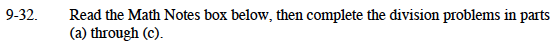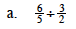Rewrite the problem with the reciprocal fraction, as shown in the Math Notes box.

$\left(\frac{6}{5}\right)\left(\frac{2}{3}\right)$

Simplify the new problem.

$\frac{(6)(2)}{(5)(3)}=\frac{12}{15}=\frac{4}{5}$

$\frac{4}{5}$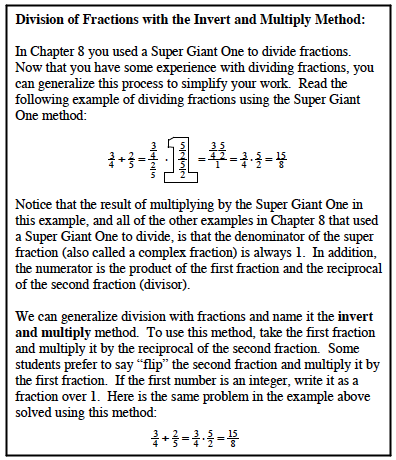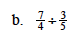Follow the same steps as in part (a).
Refer to the Math Notes box for more help.

$2\frac{11}{12}$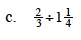Change the mixed number to a fraction greater than one before you follow the steps from part (a).